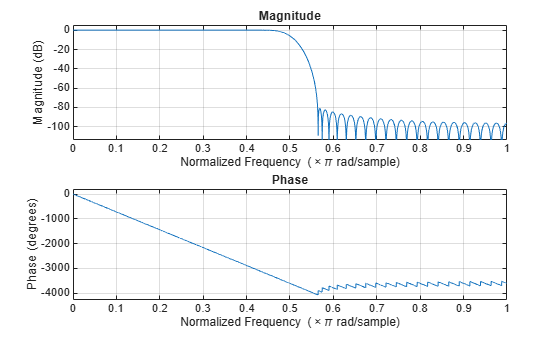freqz

Frequency response of discrete-time filter System object

Description

[h,w] = freqz(sysobj) returns the complex frequency response h of the filter System object™, sysobj. The vector w contains the frequencies (in radians/sample) at which the function evaluates the frequency response. The frequency response is evaluated at 8192 points equally spaced around the upper half of the unit circle.

[h,w] = freqz(sysobj,n) returns the complex frequency response of the filter System object and the corresponding frequencies at n points equally spaced around the upper half of the unit circle.

freqz uses the transfer function associated with the filter to calculate the frequency response of the filter with the current coefficient values.

[h,w] = freqz(sysobj,'Arithmetic',arithType) analyzes the filter System object, based on the arithmetic specified in arithType, using either of the previous syntaxes.

example

freqz(sysobj) uses fvtool to plot the magnitude and unwrapped phase of the frequency response of the filter System object sysobj.

For more input options, see freqz in Signal Processing Toolbox™.

Examples

collapse all

This examples plot the frequency response of the lowpass FIR filter using freqz.

b = fir1(80,0.5,kaiser(81,8));
firFilt = dsp.FIRFilter('Numerator',b);
freqz(firFilt);Input Arguments

collapse all

Number of points over which the frequency response is computed. For an FIR filter where n is a power of two, the computation is done faster using FFTs.

Data Types: single | double | int8 | int16 | int32 | int64 | uint8 | uint16 | uint32 | uint64

Arithmetic used in the filter analysis, specified as 'double', 'single', or 'Fixed'. When the arithmetic input is not specified and the filter System object is unlocked, the analysis tool assumes a double-precision filter. When the arithmetic input is not specified and the System object is locked, the function performs the analysis based on the data type of the locked input.

The 'Fixed' value applies to filter System objects with fixed-point properties only.

When the 'Arithmetic' input argument is specified as 'Fixed' and the filter object has the data type of the coefficients set to 'Same word length as input', the arithmetic analysis depends on whether the System object is unlocked or locked.

• unlocked –– The analysis object function cannot determine the coefficients data type. The function assumes that the coefficients data type is signed, has a 16-bit word length, and is auto scaled. The function performs fixed-point analysis based on this assumption.

• locked –– When the input data type is 'double' or 'single', the analysis object function cannot determine the coefficients data type. The function assumes that the data type of the coefficients is signed, has a 16-bit word length, and is auto scaled. The function performs fixed-point analysis based on this assumption.

To check if the System object is locked or unlocked, use the isLocked function.

When the arithmetic input is specified as 'Fixed' and the filter object has the data type of the coefficients set to a custom numeric type, the object function performs fixed-point analysis based on the custom numeric data type.

Output Arguments

collapse all

Complex n-element frequency response vector. If n is not specified, the function uses a default value of 8192. The frequency response is evaluated at n points equally spaced around the upper half of the unit circle.

Data Types: double
Complex Number Support: Yes

Frequency vector of length n, in radians/sample. w consists of n points equally spaced around the upper half of the unit circle (from 0 to π radians/sample). If n is not specified, the function uses a default value of 8192.

Data Types: double

Tips

There are several ways of analyzing the frequency response of filters. freqz accounts for quantization effects in the filter coefficients, but does not account for quantization effects in filtering arithmetic. To account for the quantization effects in filtering arithmetic, refer to function noisepsd.

Algorithms

freqz calculates the frequency response for a filter from the filter transfer function Hq(z). The complex-valued frequency response is calculated by evaluating Hq(ejω) at discrete values of w specified by the syntax you use. The integer input argument n determines the number of equally-spaced points around the upper half of the unit circle at which freqz evaluates the frequency response. The frequency ranges from 0 to π radians per sample when you do not supply a sampling frequency as an input argument. When you supply the scalar sampling frequency fs as an input argument to freqz, the frequency ranges from 0 to fs/2 Hz.